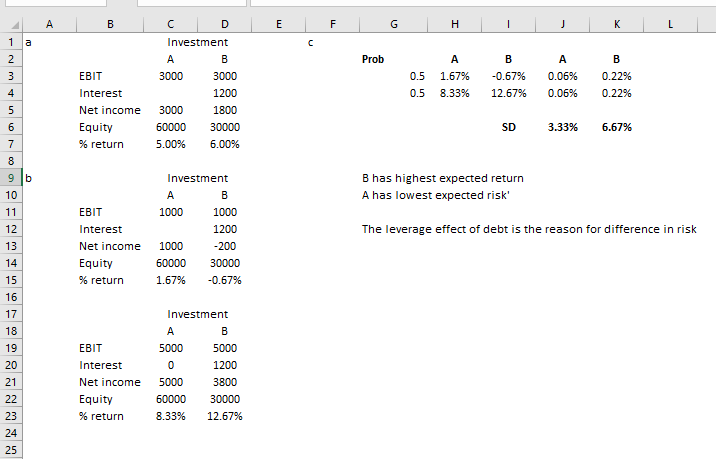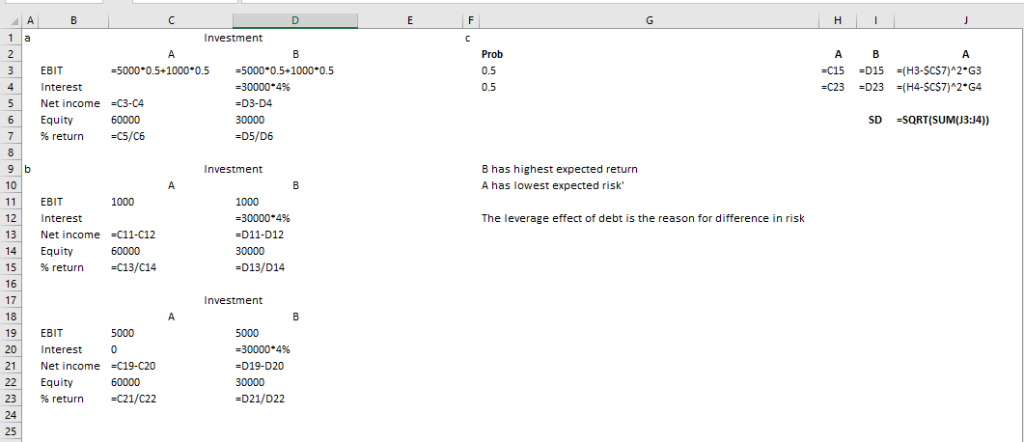Homework Help Question & Answers

# Consider two \$60,000 investments – call them Investment A and Investment B. Both investments will...

Consider two \$60,000 investments – call them Investment A and Investment B. Both investments will earn \$5,000 with a probability of 0.5 and \$1,000 with a probability of 0.5. Investment A will use 100% equity financing (issuing stocks). Investment B will get \$30,000 through issuing stocks and \$30,000 through issuing bonds. Investment B must pay 4% interest on the bonds.

a. Calculate the expected returns on equity (returns after interest payments divided by the amount of equity) for Investment A and Investment B. Express the returns as a percentage.

b. If the investments earned the lower amount (\$1,000), what is the rate of return on equity for Investment A and Investment B? If the investments earned the higher amount (\$5,000), what is the return on equity for Investment A and Investment B?

c. Using your answers from ‘a’ and ‘b’, what is the standard deviation of the rate of return on equity in each case? Which investment has the highest expected returns on equity? Which has the lowest risk? What explains the difference in risk between the two investments?

#### Homework Answers

ReportAnswer #1Add Homework Help Answer
##### Add Answer of: Consider two \$60,000 investments – call them Investment A and Investment B. Both investments will...
Your Answer: Your Name: What's your source?
Not the answer you're looking for? Ask your own homework help question. Our experts will answer your question WITHIN MINUTES for Free.
More Homework Help Questions Additional questions in this topic.

• #### The prices of 7 race cars are 126,000 140,000 140,000 180,000 400,000 400,000 819,000 The mean is \$315,000

Need Online Homework Help?

Get FREE EXPERT Answers
WITHIN MINUTES
Related Questions